Courses

# Probability - Examples (with Solutions), Quantitative Aptitude Quant Notes | EduRev

## GMAT : Probability - Examples (with Solutions), Quantitative Aptitude Quant Notes | EduRev

The document Probability - Examples (with Solutions), Quantitative Aptitude Quant Notes | EduRev is a part of the GMAT Course General Aptitude for GATE 2020.
All you need of GMAT at this link: GMAT

TERMINOLOGY

Deterministic Experiment: is the one, which gives a certain definite result. e.g. acid is added to a base.

Random Experiment: is the one which gives one or more results under identical conditions. E.g. a coin is
tossed.

The set of all possible outcomes of a random experiment is known as the sample space and every outcome
is a sample point.

Probability of an Event
If there are n-elementary events associated with a random experiment and m of them are favorable to an
event A, then the probability of happening of A is denoted by P (A) and is defined as the ratio m/n.

Probability of an event occurring =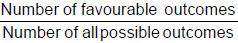Thus, P (A) =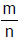.
Clearly, 0 ≤ m ≤ n, therefore 0 ≤≤ 1, so that 0 ≤ P (A) ≤ 1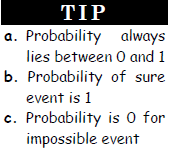Complement of an event
Since the number of cases in which the event A will not happen is n - m, therefore if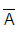denotes not happening of A, then the probability P () of not happening of A is given by
P () =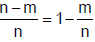= 1 – P (A)        or      P(A) + P () = 1

Ex.1 What is the probability that both the two dice thrown show a number multiple of 3?

Sol. There are only two numbers divisible by 3 (3, 6) in a dice.
Favorable chances = 2
Hence probability of a dice showing number divisible by 3 =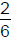=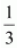Probability that both the dices show number divisible by 3 & 6 =×=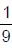The odds in favour of occurrence of the event A are defined by m: n – m i.e., P (A): P () and the odds
against the occurrence of A are defined by n-m: m, i.e., P (): P (A).

Ex.2 A spinner has 4 equal sectors colored yellow, blue, green, and red. What are the chances of
landing on blue after spinning the spinner?

Sol. This problem asked us to find the probability that the spinner will
land on blue. Let's look at some definitions and examples relating
to the problem above.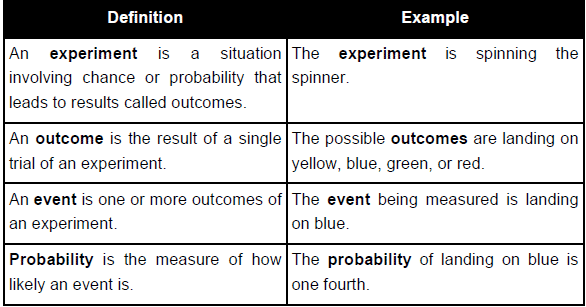Impossible event and certain event
An impossible event has no chance of occurring. If event A is impossible, then P(A) = 0
A certain event is certain to occur. If event A is certain, then P(A) = 1.

Mutually Exclusive Events
Two or more events are said to be mutually exclusive these events cannot occur simultaneously. Two or
more events are said to be compatible if they can occur simultaneously.

Two events (A and B) are mutually exclusive if the intersection of two events is null or they have no common
element i.e. A ∩ B = φ .

e.g. In drawing a card from a deck of 52 cards:
A: The event that it is red.
B: The event that it is black.
C: The event that it is a king.

In the above case events A and B are mutually exclusive but the events B and C are not mutually exclusive or
disjoint since they may have common outcomes.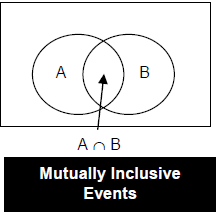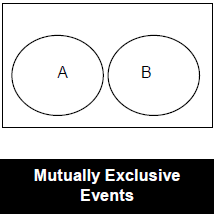Sum Rule

If E and F are two mutually exclusive events, then the probability that either event E or event F will occur in a
single trial is given by :

P(E or F) or P (E ∪ F) = P(E) + P(F)

If the event is not mutually exclusive, then
P(E ∪ F) = P(E) + P(F) – P(E and F together )

Note: P (neither E nor F) = 1 – P(E or F).

Ex.3 The probability of two events A and B are 0.25 and 0.50 respectively. The probability of their simultaneous occurrence is 0.14. Find the probability that neither A nor B occurs.

Sol. We have P(A) = 0.25, P(B) = 0.50 and P(A ∩ B) = 0.14.
Required probability =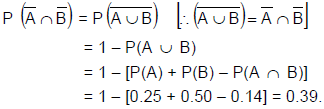Independent/ Dependent events

Two events, A and B, are independent if the fact that A occurs does not affect the probability of B occurring.

Two events, A and B, are dependent if the fact that A occurs affects the probability of B occurring.

Some other examples of independent events are:

• Tossing a coin and landing on heads, and rolling a die and getting a 5.

• Choosing a card from a deck of cards and getting a three, replacing it,
and choosing a second card and getting an ace.

• Rolling a die and getting a 4, and then rolling a second die and getting a 1.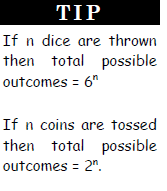Multiplication Rule

When two events, A and B, are independent, the probability of both occurring is:
P(A and B) = P(A ∩ B) = P(A) × P(B)

Ex.4 A coin is tossed and a single 6-sided dice is rolled. Find the probability of getting ahead on the
coin and a 3 on the dice.

Sol. P(head) =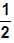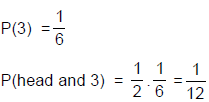Binomial Distribution: If n trials are performed under the same condition and probability of success in
each trial is p and q = 1 – p then the probability of exactly r successes in n trials is: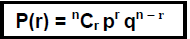Ex.5 A dice is tossed 5 times. What is the probability that 5 shows up exactly thrice?

Sol. Here the 'random experiment' consists of tossing a die 5 times and observing the number '5' as success, then p = Probability of getting '5' with single dice =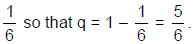Since the value of p is constant for each dice and the trials are independent, using formula for Binomial probability law, the probability of r successes is given by :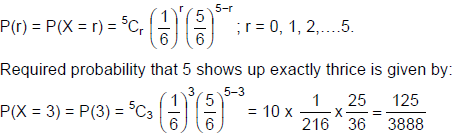Question 1:An unbiased dice is thrown. What is the probability of getting
(i) an even number;
(ii) a multiple of 3;
(iii) an even number or a multiple of 3;
(iv) an even number & a multiple of 3?
Choose the most appropriate option.

Ex.6 What is the chance of drawing an ace from a deck of cards?

Sol. There are 52 cards in a deck of cards, the total number of possible outcomes of drawing a card is 52. Since there are 4 aces in a pack of cards, the total number of favorable outcomes is 4. The chance or probability of drawing an ace from the given pack is the ratio of total number of favorable outcomes to the total number of possible outcomes. Which in this case is:Ex.7 One card is drawn from a pack of 52 cards, each of the 52 cards being equally likely to be
drawn. Find the probability that the card drawn is:
(i) an ace,

(ii) red,
(iii) either red or king,

(iv) red and a king

Sol. Out of 52 cards, one card can be drawn in 52C1 ways.
Therefore exhaustive number of cases = 52C1 = 52.
(i) There are four aces in a pack of 52 cards, out of which one can be drawn in 4C1.
Therefore Favourable number of cases = 4C1 = 4.
So, required probability =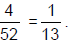(ii) There are 26 red cards, out of which one red card can be drawn in 26C1 ways. Therefore,
Favourable number of cases = 26C1 = 26.
So, required probability =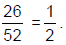(iii) There are 26 red cards including 2 red kings and there are 2 more kings. Therefore, there are 28 cards, one can be drawn in 28C1 ways.
Therefore favourable number of cases = 28C1 = 28.So, required probability =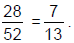(iv) There are 2 cards, which are red and king. Therefore, favourable number of cases = 2C1 = 2.

So, required probability =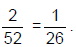Ex.8 Harshad and Amit throw with two dice. If Harshad throws 10, what is Amit’s chance of throwing a higher number?

Sol. Total number of outcomes of throwing two dice is 6 × 6 = 36.
Amit must throw either 11 or 12.
This can be done in 3 ways namely (6 + 5, 5 + 6, 6 + 6)
Thus Amit’s chance of throwing a higher number is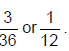Summary

The probability of an event is a measure of the chance that the event will occur as a result of an experiment.

The probability of an event A is the number of ways event A can occur divided by the total number of possible outcomes. The probability of an event A, symbolized by P(A), is a number between 0 and 1, inclusive, that measures the likelihood of an event in the following way:

• If P(A) > P(B) then event A is more likely to occur than event B.

• If P(A) = P(B) then events A and B are equally likely to occur.

• Two events are mutually exclusive if the intersection of two events is null (A ∩ B = φ )

• If E and F two events then P(E U F) = P(E) + P(F) - P(E and F together )

• If an event E is sure to occur, we say that the probability of event E is equal to 1 and we write P (E) = 1. Such events are known as certain events.

• If an event E is sure not to occur, we say that the probability of the Event E is equal to 0 and we write P(E) = 0. Such events are known as impossible events.

• The probability of E not occurring, denoted by P(not E), is given by P(not E) or P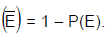• The odds in favor of occurrence of the event A are defined by m: n – m i.e., P (A): P () and the odds against the occurrence of A are defined by n – m : m, i.e., P (): P (A)

• To find the probability of two or more independent events occurring in sequence, find the probability of each event occurring separately, and then multiply the answers.

Ex.9 If a two digit no. is selected at random. What is the probability of getting a number, which has both the digits same?

Sol. total 2 digit numbers are 10 to 99 ⇒ 90
Required numbers are 11, 22, - - - - - 99 ⇒ 9
So probability =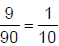Ex.10 If a number is selected at random from the 2 digit 6 multiples, what is the probability that it is divisible by 9?

Sol. Total 6 multiples = 12, - - - -, 96 ⇒ 15.
Required numbers are both multiples of 6 & 9
i. e, the multiples of 18. Those are 18, 36,- - - -, 90, total 5.       ∴ Ans =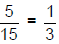Question 2:If 5 coins are tossed, what is the probability of getting at least 3 heads.

Ex.11 If 2 dies are thrown, what is the probability of getting both the numbers prime?

Sol. P (Prime on first die) × P(Prime on second die)
=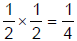Ex.12 If 3 dies are thrown, what is the probability of getting a sum 4.

Sol. The possibility is 1, 1, 2 or 1, 2, 1 or 2, 1, 1.
Ans =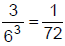Ex.13 If 2 cards are drawn from a pack, what is the probability that both the cards are of different colours?

Sol. The possibility is 1 black and 1 Red.
i.e,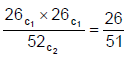Ex.14 10% of the bulbs manufactured by a company are defective. If 2 bulbs are chosen at random, what is the probability that at least one bulb is non defective.

Sol. The answer is 1 – P (Probability of getting both defective)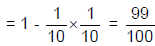Ex.15 There are 5 red, 4 green, 3 yellow & 8 white balls in a bag, If three balls are choosen at random without replacement, what is the probability that they are of same color.

Sol. All the three can be red or green or yellow or white.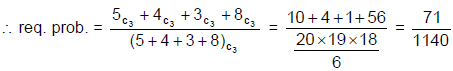Question 3:There are 4 red & 5 green balls in bag A and 5 red & 6 green balls in bag B. If a bag is selected at random and a ball is selected from that, what is the probability that it is red?

Ex.16 In a game, there are three rounds; the probabilities of winning first, second & third rounds are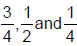respectively. A prize will be given, if any one wins all the rounds. What is the probability of winning the prize?

Sol. All the rounds have to be won to get the prize. So, the required answer is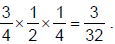Offer running on EduRev: Apply code STAYHOME200 to get INR 200 off on our premium plan EduRev Infinity!

## General Aptitude for GATE 2020

59 videos|47 docs|108 tests

,

,

,

,

,

,

,

,

,

,

,

,

,

,

,

,

,

,

,

,

,

,

,

,

;